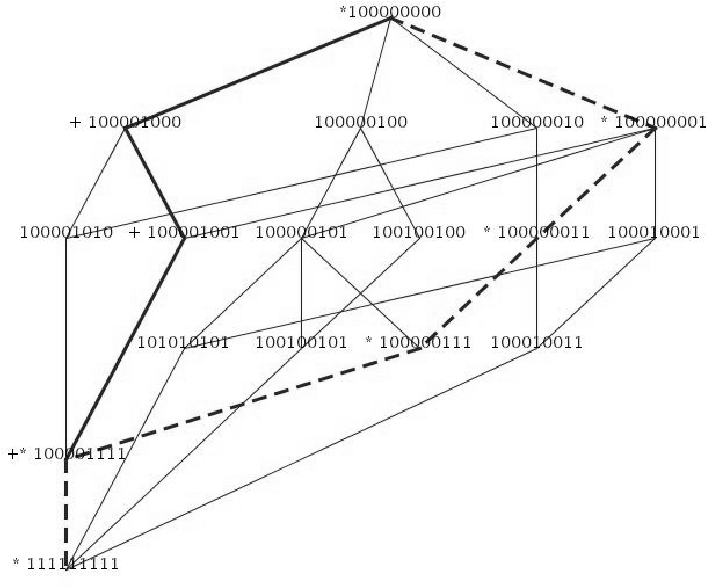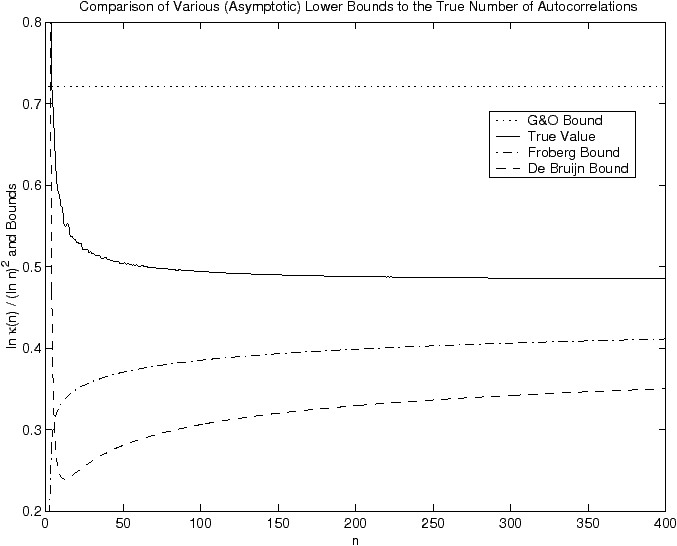https://oeis.org/

# Autocorrelation of Strings A comment on entries A005434 and A045690 of the Encyclopedia of Integer Sequences

### Eric Rivals LIRMM (Computer Science Department) CNRS - Université de Montpellier Francerivals_AT_lirmm.frhttp://www.lirmm.fr/~rivals

This page is also available in PDF format here and the corresponding publication on periods in strings is there. It also concerns entries A018819 and A000123 of the Encyclopedia of Integer Sequences .

Strings, also called word, or sequence of characters may "overlap" themselves. Example 0.1 shows how the word "abracadabra" can overlap itself.

Example 1   The word ABRACADABRA admits {0,7,11} as periods set and v := 100000010001 as autocorrelation.

 Offset: 0 1 2 3 4 5 6 7 8 9 0 A B R A C A D A B R A ⋮ ⋮ A B R A C A D A B R A ⋮ A B R A C A D A B R A Autocorrelation: 1 0 0 0 0 0 0 1 0 0 1

Example 2   The word ababababa admits {0,2,4,6,8} as periods set and has autocorrelation v := 101010101.
 Offset: 0 1 2 3 4 5 6 7 8 Word a b a b a b a b a Period 2 a b a b a b a Period 4 a b a b a Period 6 a b a Period 8 a Autocorrelation 1 0 1 0 1 0 1 0 1

An offset at which a word can overlap itself is called a period. Note that 0 is always a period and that the maximum period is n−1 if the word is of length n. Therefore, any word of length n has a non-empty set of periods, which is a subset of [0,n−1]. The set of period can be denoted as a set, but also as a binary vector of length n indexed from 0 until n−1 in which an entry equals 1 if an integer is a period of the word and 0 otherwise. This binary vector is called the autocorrelation of the word and has the same length as the word. Not all possible binary vector of length n are autocorrelation . Examples  0.1 and  0.2 illustrate these definitions.

This page summarizes some of the results published in  about the nature of autocorrelations and the number κn of different autocorrelations of words of size n (where n is any positive integer). The sequence (κn)n>0 corresponds to the sequence A005434 in the Encyclopedia of Integer Sequences (EOIS) .

For instance, it exhibits the relation between the number of binary partitions of an integer n (sequence A018819 In the EOIS ) and the number of autocorrelation of length n. The first study of autocorrelation was published in the seminal article of Guibas and Odlyzko in 1981 .

## 1  Notation, Definitions and Elementary Properties

Let Σ be a finite alphabet of size σ. A sequence of n letters of Σ indexed from 0 to n−1 is called a word or a string of length n over Σ. We denote the length of a word U:= U0U1... Un−1 by |U|. We denote by Σ*, respectively by Σn, the set of all finite words, resp. of all words of length n, over Σ.

Definition 1   Let U ∈ Σn and let p be a non-negative integer with p<n. Then p is a period of U iff the suffix of length np of U is equal to its prefix of length np. The basic period of U is its smallest non-null period, if it exists.

We denote the set of all periods of U by P(U). The autocorrelation v of U is a representation of P(U). It is a binary vector of length n such that: ∀   0 ≤ i < n, vi=1 iff iP(U), and vi=0 otherwise.

Let Γn := { v ∈ {0,1}n  |  ∃ U ∈ Σn: v = P(U) } be the set of all autocorrelations of strings in Σn. We denote its cardinality by κn. The autocorrelations in Γn can be partitioned according to their basic period; thus, for 0≤ p < n, we denote by Γn,p the subset of autocorrelations whose basic period is p, and by κn,p the cardinality of this set. The set inclusion defines a partial order on elements of Γn.

## 2  Structural Properties of Γn

First we have shown that Γn equipped with the set inclusion (denoted ⊆) is a lattice. However, for n > 6, Γn does not satisfy the Jordan-Dedekind condition. The structure is illustrated  1.Figure 1: A representation of the lattice Γ(9). The bold-edges and dashed-edges paths shows two maximal chains of different lengths between 111111111 and 100000000. The correlations on these paths are marked with a + or a *, respectively.

Moreover, we have also noticed that the complete set of periods is redundant and have defined the Irreducible Periods Set, which is the smallest subset of the periods set whose enable to recompute the periods set. There is a one-to-one (bijective) correspondance between periods sets and Irreducible Periods Sets.

## 3  Enumeration of all Autocorrelations of Length n

Guibas and Odlyzko gave a predicate Ξ that determine in linear time if a binary vector is a true autocorrelation . We build on the predicate Ξ to exhibit an enumaration algorithm for all autocorrelations. The algorithm is detailed in . Here, we provide a C and a C++ implementation of this algorithm. The C++ implementation used bitstring to store the binary vectors and is thus able to enumerate the autocorrelations until n=450 (this depends on the amount of main memory available on the computer).

Feel free to contact us per email rivals_AT_lirmm.frif you need other versions (Mac OSX or Windows), or if you want the set of autocorrelations for a given n.

## 4  Bounds on the Number of Autocorrelations

We investigated how the number κn of different autocorrelations of length n grows with n. From the characterization of autocorrelation , we know that κn is independent of the alphabet size. In , it is shown that as n→∞,
1
2ln2
+o(1) ≤
lnκn
(lnn)2
1
2ln(3/2)
+o(1).     (1)
As shown in Figure 2, these bounds are rather loose. In fact, for small n, the actual value of κn is below its asymptotic lower bound. While we conjecture that limn→∞lnκn/(lnn)2 = 1/2 ln2, it remains an open problem to derive a tight upper bound and prove this conjecture. Our contribution is that a good lower bound for κn is closely related to the number of binary partitions of an integer. Both improved bounds we derive from this relationship are also shown in Figure 2.Figure 2: True values of lnκn/(lnn)2 for n≤ 400, compared to Guibas & Odlyzko's (G&O) asymptotic lower bound, the improved asymptotic bound from Theorem 1 (ii) derived from DeBruijn's results, and the non-asymptotic lower bound from Theorem 1 (i) based on Fröberg's work. Both of these bounds converge to the G&O asymptotic value of 1/(2 ln2) for n→∞. The upper bound of G&O, corresponding to the line y=1/(2 ln(3/2))≈ 1.23, is not visible on the figure.

We have that the number of autocorrelations of length n, κn, is bounded by the number of autocorrelations of length n whose basic period is larger than n/2. The latter equals the sum of the κi for i going from 0 to ⌈ n/2 ⌉ −1. Thus, we define L0:=1, L1:=1, and, for n≥ 2, Ln := Σi=0n/2 ⌉ −1 Li. By induction, Ln≤ κn for all n≥ 0.

Now we consider a related sequence: the number of binary partitions Bn of an integer n≥ 0, i.e., the number of ways to write n as a sum of powers of 2 where the order of summands does not matter. For example, 6 can be written as such a sum in 6 different ways: 4+2, 4+1+1, 2+2+2, 2+2+1+1, 2+1+1+1+1, 1+1+1+1+1+1. Therefore B6=6. By convention, B0=1; furthermore B1=1.

Bn corresponds to the sequence A018819, and B2n to the sequence A000123 in the Encyclopedia of Integer Sequences .

We have shown that for n≥ 1, Ln = 1/2 ⋅ Bn+1. Then building on the results of Fröberg  and De Bruijn , which both gave approximations on the number of binary partitions, we provide two lower bounds (which we refer to as Fröberg's and De Bruijn's bounds in Figure 2) for κn, the number of autocorrelations of length n.

Theorem 1  Define F(n) as follows
F(n):=
 ∞ Σ k=0

nk
2
k(k+1)
2

k
.     (2)
Then:
1. For all n≥ 1, κn ≥ 0.31861 ⋅ F(n+1).
2. Asymptotically (with approximated constants),
lnκn
(lnn)2
1
2ln2

1−
lnlnn
lnn

 2
+
0.4139
lnn
1.47123   lnlnn
(lnn)2
+ O

1
(lnn)2

.

## 5  Computing the Size of Populations

The correlation of a string depends on its self-overlapping structure, but is not directly related to its characters. Hence, different strings share the same correlation. For instance over the alphabet {a,b}, take abbabba and babbabb. The population of a correlation v is the set of strings over Σ whose correlation is v. We wish to compute the size of the population of a given correlation, and by extension of all correlations. This corresponds to entry A045690 in the Encyclopedia of Integer Sequences .

In , Guibas and Odlyzko exhibit a recurrence linking the population sizes of a correlation and of its nested correlation. Here, we exhibit another recurrence which links the population size of an autocorrelation v to the population sizes of the autocorrelations it is included in. The recurrence depends on the number of free characters (nfc for short) of v, to be defined next.

Definition 2  The nfc of a correlation v is the maximum number of positions in a string U with P(U)=v that are not determined by the periods.

To illustrate this definition, note that a correlation represents a set of equalities between the characters of a string. For example, take v:=100001001 ∈ Γ9. A string U=u0... u8 with P(U)=v must satisfy the following set of equations: { u0 = u3 = u5 = u8, u1 = u6, u2 = u7}. Thus we can write any word U as u0 u1 u2 u0 u4 u0 u1 u2 u0 for some u0,u1,u2,u4 ∈Σ. So the nfc of v is 4.

The nfc is independent of Σ and can be computed from v alone. Given a correlation v and its length n, the algorithm NFC, computes the nfc of v. NFC follows the recursive structure of Predicate Ξ and requires Θ(n) time. Its pseudo-code is available here.

We now state our recurrence on the population sizes.
Theorem 2   Let nN and let vk be the k-th (k=1,...,κn) autocorrelation of Γn. Let ρk denote the number of free characters of vk, and Nk be its population size. We have:
Nkρk
 Σ j : vk ⊂ vj
Nj.

## References


N. G. DeBruijn. On Mahler's partition problem. Proc. Akad. Wet. Amsterdam, 51:659–669, 1948.


Carl-Erik Fröberg. Accurate estimation of the number of binary partitions. BIT, 17:386–391, 1977.


Leo J. Guibas and Andrew M. Odlyzko. Periods in strings. J. of Combinatorial Theory series A, 30:19–42, 1981.


Eric Rivals and Sven Rahmann. Combinatorics of Periods in Strings. J. of Combinatorial Theory series A, 104(1):95–113, October 2003.


N. J. A. Sloane. The On-Line Encyclopedia of Integer Sequences, 2004. Available at https://oeis.org/.

This document was translated from LATEX by HEVEA.